https://blog.zeromake.com zeromake'blog 2020-01-02T12:52:53.690Z Feed for Node.js zeromake a390720046@gmail.com https://github.com/zeromake keep codeing and thinking! https://blog.zeromake.com/favicon.ico All rights reserved 2019, zeromake<a390720046@gmail.com> <![CDATA[go-spring 使用学习]]> https://blog.zeromake.com/pages/go-spring-learn 2019-12-22T09:20:57.000Z 前言
• 最近发现了 go-spring 并且发布了 v1.0.0-beta 版。
• 看了一下感觉挺不错的，最近离职在家学习就花了一天时间学习这边记录一下

1# 拉取 go spring2$go get github.com/go-spring/go-spring@master3 4# 如果需要使用 go-spring 做 web 服务需要以下包5# go-spring-boot-starter 是使用 spring-boot 包装的支持 web 以及其它的启动器6$ go get github.com/go-spring/go-spring-boot-starter@master7# go-spring-web 则是配合 go-spring-boot-starter 使用的各种 web 框架的封装8$go get github.com/go-spring/go-spring-web@master 由于 go-spring 现在还是 beta 版，每天都有可能有一些重要更新建议拉取最新的 master 不过到了后面 go-spring 正式版也许就不需要直接手动拉取 @master 了，请自行判断。 ## 二、go-spring 项目包结构介绍¶ 1$ tree . -L 12.3├── CONTRIBUTING.md4├── LICENSE5├── README.md6├── RunAllTests.sh7├── RunCodeCheck.sh8├── RunGoDoc.sh9├── boot-starter10├── go.mod11├── go.sum12├── package-info.go13├── spring-boot14├── spring-core15├── starter-echo16├── starter-gin17└── starter-web18 196 directories, 9 files

starter 部分的暂时无视，这样一看就只剩下 spring-corespring-bootboot-starter

• spring-core 是用于 IoC 容器注入的核心库。
• spring-boot 是使用了 spring-core 构建的配置自动载入，还有注入的对象的启动和关闭的统一管理。
• boot-starter 简单启动和监听信号包装器。

## 三、一个简单 gin web 服务¶

1package main2 3import (4	SpringWeb "github.com/go-spring/go-spring-web/spring-web"5	SpringBoot "github.com/go-spring/go-spring/spring-boot"6	"net/http"7 8	_ "github.com/go-spring/go-spring/starter-gin"9	_ "github.com/go-spring/go-spring/starter-web"10)11 12func init() {13	SpringBoot.RegisterBean(new(Controller)).InitFunc(func(c *Controller) {14		SpringBoot.GetMapping("/", c.Home)15	})16}17 18type Controller struct{}19 20func (c *Controller) Home(ctx SpringWeb.WebContext) {21	ctx.String(http.StatusOK, "OK!")22}23 24func main() {25	SpringBoot.RunApplication("config/")26}
• 其中 init 方法里我们注册了一个 Controller 的空实例，这个不一定要在 init 中注册，可以在 SpringBoot.RunApplication 调用前的任意地方注册，使用 init 的原因是可以不依赖包内部方法只需要导入即可注入。
• 然后通过 InitFunc 注册路由，SpringBoot.GetMapping 是统一封装的路由挂载器
• Home(ctx SpringWeb.WebContext) 里的 SpringWeb.WebContext 则封装了请求响应操作。
• github.com/go-spring/go-spring/starter-gin 导入替换为 github.com/go-spring/go-spring/starter-echo 可以直接替换为 echo 框架。

## 六、spring-boot 加载配置注入对象¶

SpringBoot.RunApplication("config/")

spring-boot 支持不少格式的配置和命名方式，这些都不介绍了。

config/application.toml

1[spring.application]2name = "demo-config"3 4[file]5dir = "temp"

controllers/upload/upload.gocontroller 使用配置替换硬编码的保存文件夹路径, value:"${file.dir}" 对应配置文件的路径绑定。 1type Controller struct {2 File *file.Service autowire:""3 Dir string value:"${file.dir}"4}5 6func (c *Controller) Upload(ctx SpringWeb.WebContext) {7	// ……8	// 替换为注入的配置9	out := path.Join(c.Dir, f.Filename)10	// ……11}

## 八、通过 Condition 来限制 Bean 的注册来做到不同的 service 切换¶

docker-compose 这里我们用 docker 快速创建一个本地的 minio 服务。

1version: "3"2services:3  minio:4    image: "minio/minio:RELEASE.2019-10-12T01-39-57Z"5    volumes:6      - "./minio:/data"7    ports:8      - "9000:9000"9    environment:10      MINIO_ACCESS_KEY: minio11      MINIO_SECRET_KEY: minio12312    command:13      - "server"14      - "/data"

config/application.toml 添加 minio 配置

1[minio]2enable = true3host = "127.0.0.1"4port = 90005access = "minio"6secret = "minio123"7secure = false8bucket = "demo"

modules/minio/minio.go 单独的用 module 来做 minio 的客户端初始化。

1package minio2 3type MinioConfig struct {4	Enable bool   value:"${minio.enable:=true}" // 是否启用 HTTP5 Host string value:"${minio.host:=127.0.0.1}" // HTTP host6	Port   int    value:"${minio.port:=9000}" // HTTP 端口7 Access string value:"${minio.access:=}"        // Access8	Secret string value:"${minio.secret:=}" // Secret9 Secure bool value:"${minio.secure:=true}"    // Secure10	Bucket string value:"${minio.bucket:=}"11}12 13func init() {14 SpringBoot.RegisterNameBeanFn(15 // 给这个实例起个名字16 "minioClient",17 // 自动注入 minio 配置18 func(config MinioConfig) *minio.Client {19 // ……20 },21 // 前面的 0 代表参数位置，后面则是配置前缀22 "0:${}",23        // ConditionOnPropertyValue 会检查配置文件来确认是否注册24	).ConditionOnPropertyValue(25		"minio.enable",26		true,27	)28}

services/file/file.go

1func init() {2	SpringBoot.RegisterBean(new(Service)).ConditionOnMissingBean("minioClient")3}

services/minio/minio.go

#### 2.2.2. 基准如何运作¶

b.N increases in the approximate sequence; 1, 2, 3, 5, 10, 20, 30, 50, 100, and so on. The benchmark framework tries to be smart and if it sees small values of b.N are completing relatively quickly, it will increase the the iteration count faster.

b.N 以近似顺序增加；1, 2, 3, 5, 10, 20, 30, 50, 100 等。基准框架试图变得聪明，如果看到较小的 b.N 值相对较快地完成，它将更快地增加迭代次数。

note

1% go test -bench=. -cpu=1,2,4 ./examples/fib/2goos: darwin3goarch: amd644BenchmarkFib20             30000             39115 ns/op5BenchmarkFib20-2           30000             39468 ns/op6BenchmarkFib20-4           50000             40728 ns/op7PASS8ok      _/Users/dfc/devel/high-performance-go-workshop/examples/fib     5.531s

#### 2.2.3. 提高基准精度¶

fib 功能是一个稍作设计的示例-除非您编写 TechPower Web 服务器基准测试-否则您的业务不太可能会因您能够快速计算出斐波那契序列中的第 20 个数字而受到限制。但是，基准确实提供了有效基准的忠实示例。

1% go test -bench=. -benchtime=10s ./examples/fib/2goos: darwin3goarch: amd644BenchmarkFib20-8          300000             39318 ns/op5PASS6ok      _/Users/dfc/devel/high-performance-go-workshop/examples/fib     20.066s

为什么报告的总时间是 20 秒，而不是 10 秒？

1% go test -bench=Fib1 -count=10 ./examples/fib/2goos: darwin3goarch: amd644BenchmarkFib1-8         2000000000               1.99 ns/op5BenchmarkFib1-8         1000000000               1.95 ns/op6BenchmarkFib1-8         2000000000               1.99 ns/op7BenchmarkFib1-8         2000000000               1.97 ns/op8BenchmarkFib1-8         2000000000               1.99 ns/op9BenchmarkFib1-8         2000000000               1.96 ns/op10BenchmarkFib1-8         2000000000               1.99 ns/op11BenchmarkFib1-8         2000000000               2.01 ns/op12BenchmarkFib1-8         2000000000               1.99 ns/op13BenchmarkFib1-8         1000000000               2.00 ns/op

Fib(1) 基准测试大约需要 2 纳秒，方差为 +/- 2％。

Go 1.12 中的新增功能是 -benchtime 标志，现在需要进行多次迭代，例如。-benchtime=20x，它将准确地运行您的代码 benchtime 的时间。

尝试以10倍，20倍，50倍，100倍和300倍的 -benchtime 运行上面的 fib 测试。 你看到了什么？

tip

If you find that the defaults that go test applies need to be tweaked for a particular package, I suggest codifying those settings in a Makefile so everyone who wants to run your benchmarks can do so with the same settings.

### 2.3. 将基准与 Benchstat 进行比较¶

% go get golang.org/x/perf/cmd/benchstat

Benchstat 可以进行一系列基准测试，并告诉您它们的稳定性。 这是有关电池供电的 Fib(20) 示例。

1% go test -bench=Fib20 -count=10 ./examples/fib/ | tee old.txt2goos: darwin3goarch: amd644BenchmarkFib20-8           50000             38479 ns/op5BenchmarkFib20-8           50000             38303 ns/op6BenchmarkFib20-8           50000             38130 ns/op7BenchmarkFib20-8           50000             38636 ns/op8BenchmarkFib20-8           50000             38784 ns/op9BenchmarkFib20-8           50000             38310 ns/op10BenchmarkFib20-8           50000             38156 ns/op11BenchmarkFib20-8           50000             38291 ns/op12BenchmarkFib20-8           50000             38075 ns/op13BenchmarkFib20-8           50000             38705 ns/op14PASS15ok      _/Users/dfc/devel/high-performance-go-workshop/examples/fib     23.125s16% benchstat old.txt17name     time/op18Fib20-8  38.4µs ± 1%

benchstat 告诉我们平均值为 38.8 微秒，样本之间的变化为 +/- 2％。这对于电池供电来说相当不错。

• 第一次运行是最慢的，因为操作系统已降低 CPU 时钟以节省电量。
• 接下来的两次运行是最快的，因为操作系统确定这不是工作的短暂高峰，并且提高了时钟速度以尽快完成工作，从而希望能够返回睡觉。
• 其余运行是操作系统和供热生产的 BIOS 交互功耗。

#### 2.3.1. Improve Fib¶

tip

Saving the output from a benchmark run is useful, but you can also save the binary that produced it. This lets you rerun benchmark previous iterations. To do this, use the -c flag to save the test binary—​I often rename this binary from .test to .golden.

1% go test -c2% mv fib.test fib.golden

1func Fib(n int) int {2	switch n {3	case 0:4		return 05	case 1:6		return 17	case 2:8		return 19	default:10		return Fib(n-1) + Fib(n-2)11	}12}

tip

1% go test -c2% ./fib.golden -test.bench=. -test.count=10 > old.txt3% ./fib.test -test.bench=. -test.count=10 > new.txt4% benchstat old.txt new.txt5name     old time/op  new time/op  delta6Fib20-8  44.3µs ± 6%  25.6µs ± 2%  -42.31%  (p=0.000 n=10+10)

• 旧时代和新时期的方差 ±。 1-2％ 是好的，3-5％ 是可以的，大于 5％，并且您的某些样本将被认为不可靠。 比较一侧差异较大的基准时，请注意不要有所改善。
• p 值。 p 值小于 0.05 表示良好，大于 0.05 表示基准可能没有统计学意义。
• 缺少样本。benchstat 将报告它认为有效的旧样本和新样本中的多少个，有时即使您执行了 -count=10，也可能只报告了 9 个。 10％ 或更低的拒绝率是可以的，高于 10％ 可能表明您的设置不稳定，并且您可能比较的样本太少。

### 2.4. 避免基准化启动成本¶

1func BenchmarkExpensive(b *testing.B) {2        boringAndExpensiveSetup()3        b.ResetTimer() // (1)4        for n := 0; n < b.N; n++ {5                // function under test6        }7}

| 1 | 重置基准计时器 |

1func BenchmarkComplicated(b *testing.B) {2        for n := 0; n < b.N; n++ {3                b.StopTimer() // (1)4                complicatedSetup()5                b.StartTimer() // (2)6                // function under test7        }8}

| 1 | 暂停基准测试计时器 | | 2 | 恢复计时器 |

### 2.5. 基准分配¶

1func BenchmarkRead(b *testing.B) {2        b.ReportAllocs()3        for n := 0; n < b.N; n++ {4                // function under test5        }6}

1% go test -run=^$-bench=. bufio2goos: darwin3goarch: amd644pkg: bufio5BenchmarkReaderCopyOptimal-8 20000000 103 ns/op6BenchmarkReaderCopyUnoptimal-8 10000000 159 ns/op7BenchmarkReaderCopyNoWriteTo-8 500000 3644 ns/op8BenchmarkReaderWriteToOptimal-8 5000000 344 ns/op9BenchmarkWriterCopyOptimal-8 20000000 98.6 ns/op10BenchmarkWriterCopyUnoptimal-8 10000000 131 ns/op11BenchmarkWriterCopyNoReadFrom-8 300000 3955 ns/op12BenchmarkReaderEmpty-8 2000000 789 ns/op 4224 B/op 3 allocs/op13BenchmarkWriterEmpty-8 2000000 683 ns/op 4096 B/op 1 allocs/op14BenchmarkWriterFlush-8 100000000 17.0 ns/op 0 B/op 0 allocs/op tip You can also use the go test -benchmem flag to force the testing framework to report allocation statistics for all benchmarks run. 1% go test -run=^$ -bench=. -benchmem bufio2goos: darwin3goarch: amd644pkg: bufio5BenchmarkReaderCopyOptimal-8            20000000                93.5 ns/op            16 B/op          1 allocs/op6BenchmarkReaderCopyUnoptimal-8          10000000               155 ns/op              32 B/op          2 allocs/op7BenchmarkReaderCopyNoWriteTo-8            500000              3238 ns/op           32800 B/op          3 allocs/op8BenchmarkReaderWriteToOptimal-8          5000000               335 ns/op              16 B/op          1 allocs/op9BenchmarkWriterCopyOptimal-8            20000000                96.7 ns/op            16 B/op          1 allocs/op10BenchmarkWriterCopyUnoptimal-8          10000000               124 ns/op              32 B/op          2 allocs/op11BenchmarkWriterCopyNoReadFrom-8           500000              3219 ns/op           32800 B/op          3 allocs/op12BenchmarkReaderEmpty-8                   2000000               748 ns/op            4224 B/op          3 allocs/op13BenchmarkWriterEmpty-8                   2000000               662 ns/op            4096 B/op          1 allocs/op14BenchmarkWriterFlush-8                  100000000               16.9 ns/op             0 B/op          0 allocs/op15PASS16ok      bufio   20.366s

### 2.6. 注意编译器的优化¶

1const m1 = 0x55555555555555552const m2 = 0x33333333333333333const m4 = 0x0f0f0f0f0f0f0f0f4const h01 = 0x01010101010101015 6func popcnt(x uint64) uint64 {7	x -= (x >> 1) & m18	x = (x & m2) + ((x >> 2) & m2)9	x = (x + (x >> 4)) & m410	return (x * h01) >> 5611}12 13func BenchmarkPopcnt(b *testing.B) {14	for i := 0; i < b.N; i++ {15		popcnt(uint64(i))16	}17}

1% go test -bench=. ./examples/popcnt/2goos: darwin3goarch: amd644BenchmarkPopcnt-8       2000000000               0.30 ns/op5PASS</pre>

0.3 纳秒；这基本上是一个时钟周期。即使假设每个时钟周期中 CPU 可能正在运行一些指令，该数字似乎也过低。发生了什么？

To understand what happened, we have to look at the function under benchmake, popcnt. popcnt is a leaf function — it does not call any other functions — so the compiler can inline it.

1func BenchmarkPopcnt(b *testing.B) {2	for i := 0; i < b.N; i++ {3		// optimised away4	}5}

#### 2.6.1. 练习，实践¶

% go test -gcflags=-S
使用 gcflags="-l -S" 禁用内联，这可以影响程序输出

#### 2.6.2. 修复基准¶

1var Result uint642 3func BenchmarkPopcnt(b *testing.B) {4	var r uint645	for i := 0; i < b.N; i++ {6		r = popcnt(uint64(i))7	}8	Result = r9}

Because Result is public the compiler cannot prove that another package importing this one will not be able to see the value of Result changing over time, hence it cannot optimise away any of the operations leading to its assignment.

What happens if we assign to Result directly? Does this affect the benchmark time? What about if we assign the result of popcnt to _?

warning

### 2.7. 基准误差¶

for 循环对于基准测试的运行至关重要。

1func BenchmarkFibWrong(b *testing.B) {2	Fib(b.N)3}
1func BenchmarkFibWrong2(b *testing.B) {2	for n := 0; n < b.N; n++ {3		Fib(n)4	}5}

### 2.8. 分析基准¶

testing 包内置了对生成 CPU，内存和块配置文件的支持。

• -cpuprofile=$FILECPU 分析写入 $FILE.
• -memprofile=$FILE, 将内存分析写入 $FILE, -memprofilerate=N 将配置文件速率调整为 1/N.
• -blockprofile=$FILE, 将块分析写入 $FILE.

1% go test -run=XXX -bench=. -cpuprofile=c.p bytes2% go tool pprof c.p

## 3. 性能评估和性能分析¶

### 3.1. pprof¶

pprof 由两部分组成:

• runtime/pprof 每个 Go 程序内置的软件包
• go tool pprof 用于调查性能分析。

### 3.2. 性能分析文件类型¶

pprof 支持多种类型的性能分析，今天我们将讨论其中的三种:

• CPU profiling.
• Memory profiling.
• Block (or blocking) profiling.
• Mutex contention profiling.

#### 3.2.1. CPU 分析¶

CPU 分析文件是最常见的配置文件类型，也是最明显的配置文件。

#### 3.2.3. 块性能分析¶

• 在无缓冲的通道上发送或接收。
• 正在发送到完整频道，从空频道接收。
• 试图 锁定 被另一个 goroutine 锁定的 sync.Mutex

warning

### 3.4. 收集性能分析¶

Go 运行时的配置文件界面位于 runtime/pprof 包中。runtime/pprof 是一个非常基础的工具，由于历史原因，与各种配置文件的接口并不统一。

1import "github.com/pkg/profile"2 3func main() {4	defer profile.Start().Stop()5	// ...6}

### 3.5. Analysing a profile with pprof¶

% go tool pprof /path/to/your/profile

note

#### 3.5.2. CPU profiling (exercise)¶

1package main2 3import (4	"fmt"5	"io"6	"log"7	"os"8	"unicode"9)10 11func readbyte(r io.Reader) (rune, error) {12	var buf byte13	_, err := r.Read(buf[:])14	return rune(buf), err15}16 17func main() {18	f, err := os.Open(os.Args)19	if err != nil {20		log.Fatalf("could not open file %q: %v", os.Args, err)21	}22 23	words := 024	inword := false25	for {26		r, err := readbyte(f)27		if err == io.EOF {28			break29		}30		if err != nil {31			log.Fatalf("could not read file %q: %v", os.Args, err)32		}33		if unicode.IsSpace(r) && inword {34			words++35			inword = false36		}37		inword = unicode.IsLetter(r)38	}39	fmt.Printf("%q: %d words\n", os.Args, words)40}

1% go build && time ./words moby.txt2"moby.txt": 181275 words3 4real    0m2.110s5user    0m1.264s6sys     0m0.944s

1% time wc -w moby.txt2215829 moby.txt3 4real    0m0.012s5user    0m0.009s6sys     0m0.002s

#### 3.5.3. 添加 CPU 分析¶

1import (2        "github.com/pkg/profile"3)4 5func main() {6        defer profile.Start().Stop()7        // ...

1% go run main.go moby.txt22018/08/25 14:09:01 profile: cpu profiling enabled, /var/folders/by/3gf34_z95zg05cyj744_vhx40000gn/T/profile239941020/cpu.pprof3"moby.txt": 181275 words42018/08/25 14:09:03 profile: cpu profiling disabled, /var/folders/by/3gf34_z95zg05cyj744_vhx40000gn/T/profile239941020/cpu.pprof

1% go tool pprof /var/folders/by/3gf34_z95zg05cyj744_vhx40000gn/T/profile239941020/cpu.pprof2Type: cpu3Time: Aug 25, 2018 at 2:09pm (AEST)4Duration: 2.05s, Total samples = 1.36s (66.29%)5Entering interactive mode (type "help" for commands, "o" for options)6(pprof) top7Showing nodes accounting for 1.42s, 100% of 1.42s total8      flat  flat%   sum%        cum   cum%9     1.41s 99.30% 99.30%      1.41s 99.30%  syscall.Syscall10     0.01s   0.7%   100%      1.42s   100%  main.readbyte11         0     0%   100%      1.41s 99.30%  internal/poll.(*FD).Read12         0     0%   100%      1.42s   100%  main.main13         0     0%   100%      1.41s 99.30%  os.(*File).Read14         0     0%   100%      1.41s 99.30%  os.(*File).read15         0     0%   100%      1.42s   100%  runtime.main16         0     0%   100%      1.41s 99.30%  syscall.Read17         0     0%   100%      1.41s 99.30%  syscall.read

top 命令是您最常使用的命令。 我们可以看到该程序有 99％ 的时间花费在 syscall.Syscall 中，而一小部分花费在main.readbyte 中。

% go tool pprof -http=:8080 /var/folders/by/3gf34_z95zg05cyj744_vhx40000gn/T/profile239941020/cpu.pprof

• 图形模式
• 火焰图模式

#### 3.5.4. 改进¶

1func main() {2	defer profile.Start(profile.MemProfile, profile.MemProfileRate(1)).Stop()3	// defer profile.Start(profile.MemProfile).Stop()4 5	f, err := os.Open(os.Args)6	if err != nil {7		log.Fatalf("could not open file %q: %v", os.Args, err)8	}9 10	b := bufio.NewReader(f)11	words := 012	inword := false13	for {14		r, err := readbyte(b)15		if err == io.EOF {16			break17		}18		if err != nil {19			log.Fatalf("could not read file %q: %v", os.Args, err)20		}21		if unicode.IsSpace(r) && inword {22			words++23			inword = false24		}25		inword = unicode.IsLetter(r)26	}27	fmt.Printf("%q: %d words\n", os.Args, words)28}

将此修订程序的时间与 wc 比较。有多少差距？进行性能分析，看看还剩下什么。

#### 3.5.5. 内存分析¶

defer profile.Start(profile.MemProfile).Stop()

1% go run main2.go moby.txt22018/08/25 14:41:15 profile: memory profiling enabled (rate 4096), /var/folders/by/3gf34_z95zg05cyj744_vhx40000gn/T/profile312088211/mem.pprof3"moby.txt": 181275 words42018/08/25 14:41:15 profile: memory profiling disabled, /var/folders/by/3gf34_z95zg05cyj744_vhx40000gn/T/profile312088211/mem.pprof使用 pprof 确定分配来自何处。
1func readbyte(r io.Reader) (rune, error) {2        var buf byte // (1)3        _, err := r.Read(buf[:])4        return rune(buf), err5}

| 1 | 分配在这里 |

##### Alloc 对象与 inuse 对象¶

• -alloc_objects 报告进行分配的呼叫站点。
• -inuse_objects 报告在性能分析末尾可以访问的呼叫站点。

1const count = 1000002 3var y []byte4 5func main() {6	defer profile.Start(profile.MemProfile, profile.MemProfileRate(1)).Stop()7	y = allocate()8	runtime.GC()9}10 11// allocate allocates count byte slices and returns the first slice allocated.12func allocate() []byte {13	var x [][]byte14	for i := 0; i < count; i++ {15		x = append(x, makeByteSlice())16	}17	return x18}19 20// makeByteSlice returns a byte slice of a random length in the range [0, 16384).21func makeByteSlice() []byte {22	return make([]byte, rand.Intn(2^14))23}

1% go run main.go22018/08/25 15:22:05 profile: memory profiling enabled (rate 1), /var/folders/by/3gf34_z95zg05cyj744_vhx40000gn/T/profile730812803/mem.pprof32018/08/25 15:22:05 profile: memory profiling disabled, /var/folders/by/3gf34_z95zg05cyj744_vhx40000gn/T/profile730812803/mem.pprof

% go tool pprof -http=:8080 /var/folders/by/3gf34_z95zg05cyj744_vhx40000gn/T/profile891268605/mem.pprof% go tool pprof -http=:8080 /var/folders/by/3gf34_z95zg05cyj744_vhx40000gn/T/profile891268605/mem.pprof1% go test -run=XXX -bench=ClientServer$-blockprofile=/tmp/block.p net/http2% go tool pprof -http=:8080 /tmp/block.p#### 3.5.7. 线程创建分析¶ Go 1.11 (?) 增加了对操作系统线程创建的分析支持。 将线程创建分析添加到 godoc 中，并观察分析 godoc -http =：8080 -index 的结果。 #### 3.5.8. Framepointers¶ Go 1.7 已发布，并且与用于 amd64 的新编译器一起，现在默认情况下编译器启用了帧指针。 帧指针是一个始终指向当前堆栈帧顶部的寄存器。 帧指针使诸如 gdb(1)perf(1) 之类的工具能够理解 Go 调用堆栈。 在本研讨会中，我们不会介绍这些工具，但是您可以阅读和观看我的演讲，该演讲以七种不同的方式介绍 Go 程序。 #### 3.5.9. 练习¶ • 根据您熟悉的一段代码生成一个性能分析。如果您没有代码示例，请尝试对 godoc 进行分析。 1% go get golang.org/x/tools/cmd/godoc2% cd$GOPATH/src/golang.org/x/tools/cmd/godoc3% vim main.go
• 如果要在一台计算机上生成性能分析，然后在另一台计算机上检查性能分析，您将如何处理？

## 4. 编译优化¶

• 逃逸分析
• 内联
• 消除死代码

### 4.1. History of the Go compiler¶

Go 编译器大约在 2007 时作为 Plan9 编译器工具链的分支而开始的。当时的编译器与 Aho 和 Ullman 的 Dragon Book 非常相似。

### 4.2. 逃逸优化¶

Go 规范的兼容 Go 实现 可以 将每个分配存储在堆上。这将对垃圾收集器施加很大压力，但是这绝不是不正确的。多年来，gccgo 对逃逸分析的支持非常有限，因此可以有效地认为它在这种模式下运行。

1type Foo struct {2	a, b, c, d int3}4 5func NewFoo() *Foo {6	return &Foo{a: 3, b: 1, c: 4, d: 7}7}

1func Sum() int {2	const count = 1003	numbers := make([]int, count)4	for i := range numbers {5		numbers[i] = i + 16	}7 8	var sum int9	for _, i := range numbers {10		sum += i11	}12	return sum13}14 15func main() {16	answer := Sum()17	fmt.Println(answer)18}

sum 将 1 与 100 之间的 int 相加并返回结果。

#### 4.2.1. 证明它!¶

1% go build -gcflags=-m examples/esc/sum.go2# command-line-arguments3examples/esc/sum.go:22:13: inlining call to fmt.Println4examples/esc/sum.go:8:17: Sum make([]int, count) does not escape5examples/esc/sum.go:22:13: answer escapes to heap6examples/esc/sum.go:22:13: io.Writer(os.Stdout) escapes to heap7examples/esc/sum.go:22:13: main []interface {} literal does not escape8<autogenerated>:1: os.(*File).close .this does not escape

1var answer = Sum()2fmt.Println([]interface{&answer}...)

1% go build -gcflags='-m -m' examples/esc/sum.go 2>&1 | grep sum.go:222examples/esc/sum.go:22:13: inlining call to fmt.Println func(...interface {}) (int, error) { return fmt.Fprintln(io.Writer(os.Stdout), fmt.a...) }3examples/esc/sum.go:22:13: answer escapes to heap4examples/esc/sum.go:22:13:      from ~arg0 (assign-pair) at examples/esc/sum.go:22:135examples/esc/sum.go:22:13: io.Writer(os.Stdout) escapes to heap6examples/esc/sum.go:22:13:      from io.Writer(os.Stdout) (passed to call[argument escapes]) at examples/esc/sum.go:22:137examples/esc/sum.go:22:13: main []interface {} literal does not escape

#### 4.2.2. 练习¶

• 这种优化对所有 count 的值都适用吗？
• 如果 count 是变量而不是常量，此优化是否成立？
• 如果 countSum 的参数，此优化是否成立？

#### 4.2.3. Escape analysis (continued)¶

1type Point struct{ X, Y int }2 3const Width = 6404const Height = 4805 6func Center(p *Point) {7	p.X = Width / 28	p.Y = Height / 29}10 11func NewPoint() {12	p := new(Point)13	Center(p)14	fmt.Println(p.X, p.Y)15}

NewPoint creates a new *Point value, p. We pass p to the Center function which moves the point to a position in the center of the screen. Finally we print the values of p.X and p.Y.

NewPoint 创建一个新的 *Pointp。 我们将 p 传递给 Center 函数，该函数将点移动到屏幕中心的位置。 最后，我们输出 p.Xp.Y 的值。

1% go build -gcflags=-m examples/esc/center.go2# command-line-arguments3examples/esc/center.go:11:6: can inline Center4examples/esc/center.go:18:8: inlining call to Center5examples/esc/center.go:19:13: inlining call to fmt.Println6examples/esc/center.go:11:13: Center p does not escape7examples/esc/center.go:19:15: p.X escapes to heap8examples/esc/center.go:19:20: p.Y escapes to heap9examples/esc/center.go:19:13: io.Writer(os.Stdout) escapes to heap10examples/esc/center.go:17:10: NewPoint new(Point) does not escape11examples/esc/center.go:19:13: NewPoint []interface {} literal does not escape12<autogenerated>:1: os.(*File).close .this does not escape

### 4.3. 内联¶

• 如果您的函数做了很多工作，那么前导开销将可以忽略不计。这就是为什么功能要达到一定的大小（目前有一些指令，加上一些阻止全部内联的操作，例如，在 Go 1.7 之前进行切换）
• 另一方面，小的功能为执行的相对少量的有用工作支付固定的开销。这些是内联目标的功能，因为它们最大程度地受益。

#### 4.3.1. Inlining (example)¶

1func Max(a, b int) int {2	if a > b {3		return a4	}5	return b6}7 8func F() {9	const a, b = 100, 2010	if Max(a, b) == b {11		panic(b)12	}13}

1% go build -gcflags=-m examples/inl/max.go2# command-line-arguments3examples/inl/max.go:4:6: can inline Max4examples/inl/max.go:11:6: can inline F5examples/inl/max.go:13:8: inlining call to Max6examples/inl/max.go:20:6: can inline main7examples/inl/max.go:21:3: inlining call to F8examples/inl/max.go:21:3: inlining call to Max

• 第 3 行中的第一个是 Max 的声明，告诉我们可以内联。
• 第二个报告说，Max 的主体已在第 12 行内联到调用方中。

#### 4.3.2. 内联是什么样的？¶

1% go build -gcflags=-S examples/inl/max.go 2>&1 | grep -A5 '"".F STEXT'2"".F STEXT nosplit size=2 args=0x0 locals=0x03        0x0000 00000 (/Users/dfc/devel/high-performance-go-workshop/examples/inl/max.go:11)     TEXT    "".F(SB), NOSPLIT|ABIInternal, $0-04 0x0000 00000 (/Users/dfc/devel/high-performance-go-workshop/examples/inl/max.go:11) FUNCDATA$0, gclocals·33cdeccccebe80329f1fdbee7f5874cb(SB)5        0x0000 00000 (/Users/dfc/devel/high-performance-go-workshop/examples/inl/max.go:11)     FUNCDATA        $1, gclocals·33cdeccccebe80329f1fdbee7f5874cb(SB)6 0x0000 00000 (/Users/dfc/devel/high-performance-go-workshop/examples/inl/max.go:11) FUNCDATA$3, gclocals·33cdeccccebe80329f1fdbee7f5874cb(SB)7        0x0000 00000 (/Users/dfc/devel/high-performance-go-workshop/examples/inl/max.go:13)     PCDATA  $2,$0

1func F() {2    return3}

-S 的输出不是二进制文件中的最终机器代码。链接器在最终链接阶段进行一些处理。像 FUNCDATAPCDATA 这样的行是垃圾收集器的元数据，它们在链接时会移到其他位置。如果您正在读取 -S 的输出，则只需忽略 FUNCDATAPCDATA 行；它们不是最终二进制文件的一部分。

#### 4.3.3. 讨论¶

note

-gcflags=-S 不会阻止在您的工作目录中构建最终的二进制文件。如果发现随后的 go build … 运行没有输出，请删除工作目录中的 ./max 二进制文件。

#### 4.3.4. 调整内联级别¶

• -gcflags=-l, 禁用内联.
• 没有，正常内联。
• -gcflags='-l -l' 内联级别 2，更具攻击性，可能更快，可能会生成更大的二进制文件。
• -gcflags='-l -l -l' 内联 3 级，再次更具攻击性，二进制文件肯定更大，也许再次更快，但也可能有问题。
• -gcflags=-l=4 Go 1.11 中的（四个 -l）将启用实验性 mid stack inlining optimisation。我相信从 Go 1.12 开始它没有任何作用。

tip

### 4.4. 消除无效代码¶

Before:

1func Max(a, b int) int {2	if a > b {3		return a4	}5	return b6}7 8func F() {9	const a, b = 100, 2010	if Max(a, b) == b {11		panic(b)12	}13}

After:

1func F() {2	const a, b = 100, 203	var result int4	if a > b {5		result = a6	} else {7		result = b8	}9	if result == b {10		panic(b)11	}12}

1func F() {2	const a, b = 100, 203	var result int4	if true {5		result = a6	} else {7		result = b8	}9	if result == b {10		panic(b)11	}12}

1func F() {2        const a, b = 100, 203        const result = a4        if result == b {5                panic(b)6        }7}

1func F() {2        const a, b = 100, 203        const result = a4        if false {5                panic(b)6        }7}

1func F() {2        const a, b = 100, 203        const result = a4}

1func F() {2}

#### 4.4.1. 消除无效代码（续）¶

const debug = false

### 4.5. 编译器标识练习¶

% go build -gcflags=\$FLAGS

• -S 打印正在编译的程序包的 (Go 风格) 程序集。
• -l 控制内联的行为； -l 禁用内联，-l -l 增加内联（更多 -l 增加编译器对内联代码的需求）。尝试编译时间，程序大小和运行时间的差异。
• -m 控制诸如内联，转义分析之类的优化决策的打印。-m -m 打印有关编译器思想的更多详细信息。
• -l -N 禁用所有优化。

note

### 4.6. 边界检查消除¶

Go 是一种边界检查语言。这意味着将检查数组和切片下标操作，以确保它们在相应类型的范围内。

1var v = make([]int, 9)2 3var A, B, C, D, E, F, G, H, I int4 5func BenchmarkBoundsCheckInOrder(b *testing.B) {6    for n := 0; n < b.N; n++ {7        A = v8        B = v9        C = v10        D = v11        E = v12        F = v13        G = v14        H = v15        I = v16    }17}

1func BenchmarkBoundsCheckOutOfOrder(b *testing.B) {2    for n := 0; n < b.N; n++ {3        I = v4        A = v5        B = v6        C = v7        D = v8        E = v9        F = v10        G = v11        H = v12    }13}

#### 4.6.1. 练习¶

• 重新排列下标操作的顺序是否会影响函数的大小？它会影响功能的速度吗？
• 如果将 v 移入 基准 函数内部会怎样？
• 如果 v 被声明为数组，var v  int 会发生什么？

## 5. 执行追踪器¶

### 5.1. 什么是执行跟踪器，为什么需要它？¶

examples/mandelbrot 目录包含一个简单的 mandelbrot 生成器。该代码来自 Francesc Campoy’s mandelbrot package

1% cd examples/mandelbrot2% go build && ./mandelbrot#### 5.1.1. 多久时间？¶

1% time ./mandelbrot2real    0m1.654s3user    0m1.630s4sys     0m0.015s

note

### 5.2. 生成性能分析¶

1. 直接使用 runtime/pprof 软件包。
2. 使用类似 github.com/pkg/profile 的包装器自动执行此操作。

### 5.3. 使用 runtime/pprof 生成性能分析¶

1 2import "runtime/pprof"3 4func main() {5	pprof.StartCPUProfile(os.Stdout)6	defer pprof.StopCPUProfile()

By adding this code to the top of the main function, this program will write a profile to os.Stdout.

1% cd examples/mandelbrot-runtime-pprof2% go run mandelbrot.go > cpu.pprof

note

#### 5.3.1. 使用 github.com/pkg/profile 生成性能分析¶

• 如果您忘记了将输出重定向到文件，则会破坏该终端会话。😞（提示：reset(1) 是您的朋友）
• 如果您向 os.Stdout 中写入其他内容 (例如，fmt.Println)，则会破坏跟踪。

1 2import "github.com/pkg/profile"3 4func main() {5	defer profile.Start(profile.CPUProfile, profile.ProfilePath(".")).Stop()

1% go run mandelbrot.go22017/09/17 12:22:06 profile: cpu profiling enabled, cpu.pprof32017/09/17 12:22:08 profile: cpu profiling disabled, cpu.pprof

note

#### 5.3.2. 分析性能¶

% go tool pprof -http=:8080 cpu.pprof

note

1% go tool pprof cpu.pprof2Type: cpu3Time: Mar 24, 2019 at 5:18pm (CET)4Duration: 2.16s, Total samples = 1.91s (88.51%)5Entering interactive mode (type "help" for commands, "o" for options)6(pprof) top7Showing nodes accounting for 1.90s, 99.48% of 1.91s total8Showing top 10 nodes out of 359      flat  flat%   sum%        cum   cum%10     0.82s 42.93% 42.93%      1.63s 85.34%  main.fillPixel11     0.81s 42.41% 85.34%      0.81s 42.41%  main.paint12     0.11s  5.76% 91.10%      0.12s  6.28%  runtime.mallocgc13     0.04s  2.09% 93.19%      0.04s  2.09%  runtime.memmove14     0.04s  2.09% 95.29%      0.04s  2.09%  runtime.nanotime15     0.03s  1.57% 96.86%      0.03s  1.57%  runtime.pthread_cond_signal16     0.02s  1.05% 97.91%      0.04s  2.09%  compress/flate.(*compressor).deflate17     0.01s  0.52% 98.43%      0.01s  0.52%  compress/flate.(*compressor).findMatch18     0.01s  0.52% 98.95%      0.01s  0.52%  compress/flate.hash419     0.01s  0.52% 99.48%      0.01s  0.52%  image/png.filter

1(pprof) top --cum2Showing nodes accounting for 1630ms, 85.34% of 1910ms total3Showing top 10 nodes out of 354      flat  flat%   sum%        cum   cum%5         0     0%     0%     1840ms 96.34%  main.main6         0     0%     0%     1840ms 96.34%  runtime.main7     820ms 42.93% 42.93%     1630ms 85.34%  main.fillPixel8         0     0% 42.93%     1630ms 85.34%  main.seqFillImg9     810ms 42.41% 85.34%      810ms 42.41%  main.paint10         0     0% 85.34%      210ms 10.99%  image/png.(*Encoder).Encode11         0     0% 85.34%      210ms 10.99%  image/png.Encode12         0     0% 85.34%      160ms  8.38%  main.(*img).At13         0     0% 85.34%      160ms  8.38%  runtime.convT2Inoptr14         0     0% 85.34%      150ms  7.85%  image/png.(*encoder).writeIDATs

note### 5.4. 跟踪和性能分析¶

#### 5.4.1. 使用执行跟踪器¶

1 2import "github.com/pkg/profile"3 4func main() {5	defer profile.Start(profile.TraceProfile, profile.ProfilePath(".")).Stop()

1% go build mandelbrot.go2% time ./mandelbrot32017/09/17 13:19:10 profile: trace enabled, trace.out42017/09/17 13:19:12 profile: trace disabled, trace.out5 6real    0m1.740s7user    0m1.707s8sys     0m0.020s

1% go tool trace trace.out22017/09/17 12:41:39 Parsing trace...32017/09/17 12:41:40 Serializing trace...42017/09/17 12:41:40 Splitting trace...52017/09/17 12:41:40 Opening browser. Trace viewer s listening on http://127.0.0.1:57842

#### 5.4.2. 分析追踪¶

1func seqFillImg(m *img) {2	for i, row := range m.m {3		for j := range row {4			fillPixel(m, i, j)5		}6	}7}

go tool trace

• 抱歉，该工具使用 Chrome 内置的 javascript 调试支持。跟踪配置文件只能在 Chrome 中查看，而不能在 Firefox, Safari, IE/Edge 中使用。
• 因为这是 Google 产品，所以它支持键盘快捷键。使用 WASD 导航，使用 ? 获取列表。
• 查看追踪可能会占用大量内存。 认真地说，4Gb 不会削减它，8Gb 可能是最小值，更多肯定更好。
• 如果您是从 Fedora 之类的 OS 发行版中安装 Go 的，则跟踪查看器的支持文件可能不是主 golang deb/rpm 的一部分，它们可能位于某些 -extra 软件包中。 !!!

### 5.5. 使用多个 CPU¶

Mandelbrot 的生成称为 embarassingly_parallel 。每个像素彼此独立，都可以并行计算。所以，让我们尝试一下。

1% go build mandelbrot.go2% time ./mandelbrot -mode px32017/09/17 13:19:48 profile: trace enabled, trace.out42017/09/17 13:19:50 profile: trace disabled, trace.out5 6real    0m1.764s7user    0m4.031s8sys     0m0.865s

• 似乎需要完成很多工作，但是如果您放大放大，就会发现差距。据信这是调度程序。
• 虽然我们使用所有四个内核，但是由于每个 fillPixel 的工作量相对较小，因此我们在调度开销方面花费了大量时间。

### 5.6. 整理工作¶

1% go build mandelbrot.go2% time ./mandelbrot -mode row32017/09/17 13:41:55 profile: trace enabled, trace.out42017/09/17 13:41:55 profile: trace disabled, trace.out5 6real    0m0.764s7user    0m1.907s8sys     0m0.025s

• 在程序开始时，我们看到 goroutine 的数量大约为 1000 这是对上一条跟踪中看到的 1 << 20 的改进。
• 放大后，我们看到 onePerRowFillImg 运行时间更长，并且由于 goroutine 生产 工作尽早完成，调度程序有效地处理了其余可运行的 goroutine

### 5.7. 使用 workers¶

mandelbrot.go 支持另一种模式，请尝试一下

1% go build mandelbrot.go2% time ./mandelbrot -mode workers32017/09/17 13:49:46 profile: trace enabled, trace.out42017/09/17 13:49:50 profile: trace disabled, trace.out5 6real    0m4.207s7user    0m4.459s8sys     0m1.284s

1% go build mandelbrot.go2% time ./mandelbrot -mode workers -workers 432017/09/17 13:52:51 profile: trace enabled, trace.out42017/09/17 13:52:57 profile: trace disabled, trace.out5 6real    0m5.528s7user    0m7.307s8sys     0m4.311s

• 在没有 worker 处理器准备接收之前，生产者无法发送作品。
• worker 处理器要等到有人准备派遣后才能接受工作，因此他们在等待时会互相竞争。
• 发送者没有特权，它不能比已经运行的工作者享有优先权。

### 5.8. 使用缓冲通道¶

1 2import "github.com/pkg/profile"3 4func main() {5	defer profile.Start(profile.TraceProfile, profile.ProfilePath(".")).Stop()
1% go build mandelbrot.go2% time ./mandelbrot -mode workers -workers 432017/09/17 14:23:56 profile: trace enabled, trace.out42017/09/17 14:23:57 profile: trace disabled, trace.out5 6real    0m0.905s7user    0m2.150s8sys     0m0.121s

• 生产者不必等待 worker 处理器的到来，它可以迅速填补渠道。
• worker 处理器可以快速从通道中取出下一个物品，而无需休眠等待生产。

### 5.9. Mandelbrot 微服务¶

1% go run examples/mandelweb/mandelweb.go22017/09/17 15:29:21 listening on http://127.0.0.1:8080/

http://127.0.0.1:8080/mandelbrot

#### 5.9.2. 通过 http 收集跟踪¶

import _ "net/http/pprof"

warning

net/http/pprofhttp.DefaultServeMux 注册。如果您隐式或显式地使用该 ServeMux，则可能会无意间将 pprof 端点公开到 Internet。这可能导致源代码泄露。您可能不想这样做。

% curl -o trace.out http://127.0.0.1:8080/debug/pprof/trace?seconds=5

#### 5.9.3. 产生一些负载¶

% go get -u github.com/rakyll/hey

% hey -c 1 -n 1000 -q 1 http://127.0.0.1:8080/mandelbrot

1% curl -o trace.out http://127.0.0.1:8080/debug/pprof/trace?seconds=52  % Total    % Received % Xferd  Average Speed   Time    Time     Time  Current3                                 Dload  Upload   Total   Spent    Left  Speed4100 66169    0 66169    0     0  13233      0 --:--:--  0:00:05 --:--:-- 173905% go tool trace trace.out62017/09/17 16:09:30 Parsing trace...72017/09/17 16:09:30 Serializing trace...82017/09/17 16:09:30 Splitting trace...92017/09/17 16:09:30 Opening browser.10Trace viewer is listening on http://127.0.0.1:60301

#### 5.9.4. 模拟过载¶

% hey -c 5 -n 1000 -q 5 http://127.0.0.1:8080/mandelbrot

1% curl -o trace.out http://127.0.0.1:8080/debug/pprof/trace?seconds=52  % Total    % Received % Xferd  Average Speed   Time    Time     Time  Current3                                Dload  Upload   Total   Spent    Left  Speed4100 66169    0 66169    0     0  13233      0 --:--:--  0:00:05 --:--:-- 173905% go tool trace trace.out62017/09/17 16:09:30 Parsing trace...72017/09/17 16:09:30 Serializing trace...82017/09/17 16:09:30 Splitting trace...92017/09/17 16:09:30 Opening browser. Trace viewer is listening on http://127.0.0.1:60301

#### 5.9.5. 额外的信誉，Eratosthenes 的筛子¶

concurrent prime sieve 是最早编写的 Go 程序之一。

Ivan Daniluk 撰写了一篇关于可视化的很棒的文章

## 6. 内存和垃圾收集器¶

Go 是一种 gc 语言。这是设计原则，不会改变。

### 6.1. gc 的世界观¶

Go GC 的设计倾向于在 最大吞吐量降低延迟。它将一些分配成本移到了 mutator 上，以减少以后的清理成本。

### 6.2. 垃圾收集器设计¶

• Go 1.0, 停止大量基于 tcmalloc 的世界标记清除收集器。
• Go 1.3, 完全精确的收集器，不会将堆上的大数字误认为是指针，从而不会浪费内存。
• Go 1.5, 新的 GC 设计，着重于 吞吐量 延迟
• Go 1.6, GC 的改进，以较低的延迟处理较大的堆。
• Go 1.7, 较小的 GC 改进，主要是重构。
• Go 1.8, 进一步工作以减少 STW 时间，现在已降至 100 微秒范围。
• Go 1.10+, 摆脱纯粹的合作 Goroutine 调度 以降低触发整个GC周期时的延迟。

### 6.3. 垃圾收集器监控¶

1% env GODEBUG=gctrace=1 godoc -http=:80802gc 1 @0.012s 2%: 0.026+0.39+0.10 ms clock, 0.21+0.88/0.52/0+0.84 ms cpu, 4->4->0 MB, 5 MB goal, 8 P3gc 2 @0.016s 3%: 0.038+0.41+0.042 ms clock, 0.30+1.2/0.59/0+0.33 ms cpu, 4->4->1 MB, 5 MB goal, 8 P4gc 3 @0.020s 4%: 0.054+0.56+0.054 ms clock, 0.43+1.0/0.59/0+0.43 ms cpu, 4->4->1 MB, 5 MB goal, 8 P5gc 4 @0.025s 4%: 0.043+0.52+0.058 ms clock, 0.34+1.3/0.64/0+0.46 ms cpu, 4->4->1 MB, 5 MB goal, 8 P6gc 5 @0.029s 5%: 0.058+0.64+0.053 ms clock, 0.46+1.3/0.89/0+0.42 ms cpu, 4->4->1 MB, 5 MB goal, 8 P7gc 6 @0.034s 5%: 0.062+0.42+0.050 ms clock, 0.50+1.2/0.63/0+0.40 ms cpu, 4->4->1 MB, 5 MB goal, 8 P8gc 7 @0.038s 6%: 0.057+0.47+0.046 ms clock, 0.46+1.2/0.67/0+0.37 ms cpu, 4->4->1 MB, 5 MB goal, 8 P9gc 8 @0.041s 6%: 0.049+0.42+0.057 ms clock, 0.39+1.1/0.57/0+0.46 ms cpu, 4->4->1 MB, 5 MB goal, 8 P10gc 9 @0.045s 6%: 0.047+0.38+0.042 ms clock, 0.37+0.94/0.61/0+0.33 ms cpu, 4->4->1 MB, 5 MB goal, 8 P

DEMO: 显示启用了 GODEBUG=gctrace=1godoc

tip

import _ "net/http/pprof"

• A list of all the running goroutines, /debug/pprof/heap?debug=1.
• A report on the memory allocation statistics, /debug/pprof/heap?debug=1.

note

net/http/pprof 将使用默认的 http.ServeMux 注册自己。

DEMO: godoc -http=:8080, 会显示 /debug/pprof

#### 6.3.1. 垃圾收集器调整¶

Go 运行时提供了一个用于调整 GC 的环境变量 GOGC

GOGC 的公式是

goal = reachabl\e * (1 + (GOGC)/100)

$512MB = 256MB * (1 + 100/100)$

• GOGC 的值大于100会使堆增长更快，从而减轻了 GC 的压力。
• 小于 100 的 GOGC 值会导致堆缓慢增长，从而增加了 GC 的压力。

### 6.4. 减少分配¶

1func (r *Reader) Read() ([]byte, error)2func (r *Reader) Read(buf []byte) (int, error)

### 6.5. strings 和 []bytes¶

bytes 包包含许多与 strings 软件包相同的操作 - Split, Compare, HasPrefixTrim 等。

### 6.6. 使用 []byte 作为 map 的 key¶

1var m map[string]string2v, ok := m[string(bytes)]

1key := string(bytes)2val, ok := m[key]

### 6.7. 避免字符串连接¶

1s := request.ID2s += " " + client.Addr().String()3s += " " + time.Now().String()4r = s
1var b bytes.Buffer2fmt.Fprintf(&b, "%s %v %v", request.ID, client.Addr(), time.Now())3r = b.String()
r = fmt.Sprintf("%s %v %v", request.ID, client.Addr(), time.Now())
1b := make([]byte, 0, 40)2b = append(b, request.ID...)3b = append(b, ' ')4b = append(b, client.Addr().String()...)5b = append(b, ' ')6b = time.Now().AppendFormat(b, "2006-01-02 15:04:05.999999999 -0700 MST")7r = string(b)
1var b strings.Builder2b.WriteString(request.ID)3b.WriteString(" ")4b.WriteString(client.Addr().String())5b.WriteString(" ")6b.WriteString(time.Now().String())7r = b.String()

DEMO: go test -bench=. ./examples/concat

### 6.8. 如果长度已知，则预分配片¶

1func main() {2	b := make([]int, 1024)3	b = append(b, 99)4	fmt.Println("len:", len(b), "cap:", cap(b))5}

Before

1var s []string2for _, v := range fn() {3        s = append(s, v)4}5return s

After

1vals := fn()2s := make([]string, len(vals))3for i, v := range vals {4        s[i] = v5}6return s

### 6.9. 使用 sync.Pool¶

sync 软件包带有 sync.Pool 类型，用于重用公共对象。

sync.Pool 没有固定大小或最大容量。您添加到它并从中取出直到发生 GC，然后将其无条件清空。这是 by design:

1var pool = sync.Pool{2    New: func() interface{} {3        return make([]byte, 4096)4    },5}6 7func fn() {8	buf := pool.Get().([]byte) // takes from pool or calls New9	// do work10	pool.Put(buf) // returns buf to the pool11}

warning

sync.Pool 不是缓存。它可以并且将在任何时间清空。

tip

Go 1.13 中可能会更改在每个 GC 上清空的 sync.Pool 的设计，这将有助于提高其实用性。

### 6.10. 练习¶

• 使用 godoc（或其他程序）观察使用 GODEBUG=gctrace=1 改变 GOGC 的结果。
• 使用 bytes, string 作为 map key 并检查基准。
• 不同字符串连接策略的基准分配。

## 7. 提示和旅行¶

### 7.1. Goroutines¶

Goroutines 是使其非常适合现代硬件的关键功能。

Goroutines 易于使用，而且创建成本低廉，您可以将它们视为 几乎 免费。

Go 运行时已针对具有成千上万个 goroutine 作为标准的程序而编写，数十万个并不意外。

2048 * 1,000,000 个 goroutines == 2GB 的内存，他们还没有做任何事情。

#### 7.1.1. 知道何时停止 goroutine¶

Goroutine 的启动方便，运行也很方便，但是在内存占用方面确实有一定的成本。您不能创建无限数量的它们。

tip

### 7.2. Go 对某些请求使用有效的网络轮询¶

Go 运行时使用有效的操作系统轮询机制（kqueue，epoll，windows IOCP等）处理网络IO。一个单一的操作系统线程将为许多等待的 goroutine 提供服务。

tip

1var semaphore = make(chan struct{}, 10)2 3func processRequest(work *Work) {4	semaphore <- struct{}{} // acquire semaphore5	// process request6	<-semaphore // release semaphore7}

### 7.3. 注意您的应用程序中的 IO multipliers¶

• 每个传入请求的 IO 请求数量；单个客户端请求生成多少个IO事件？它可能平均为 1，或者如果从缓存中提供了许多请求，则可能小于一个。
• 服务查询所需的读取量；它是固定的，N + 1还是线性的（读取整个表以生成结果的最后一页）。

### 7.6. defer 消耗很大，还是？¶

defer 消耗很高，因为它必须记录下 defer 的论点。

defer mu.Unlock()

1defer func() {2    mu.Unlock()3}()

### 7.7. 避免 Finalisers¶

Finalisation 是一种将行为附加到即将被垃圾回收的对象的技术。

finalizer 是 gc 周期的一部分，这意味着它们何时运行将是不可预测的，并且与减少 gc 操作的目标相矛盾。

### 7.8. 减少 cgo¶

cgo 允许 Go 程序调用 C 库。

C 代码和 Go 代码生活在两个不同的世界中，cgo 穿越了它们之间的边界。

cgo 调用类似于阻塞 IO，它们在操作期间消耗线程。

#### 7.8.1. 其实，也许避免 cgo¶

cgo 的开销很高。

• 如果C代码花费很长时间，则 cgo 开销并不重要。
• 如果您使用 cgo 调用非常短的 C 函数（其开销最明显），请在 Go 中重写该代码 - 根据定义，这很短。
• 如果您在紧密的循环中使用了大量昂贵的 C 代码，那么为什么要使用 Go？

### 7.9. 始终使用最新发布的Go版本¶

• Go 1.4 不应该使用。
• Go 1.5 和 1.6 编译器速度较慢，但生成的代码更快，GC更快。
• Go 1.7 与 1.6 相比，编译速度提高了约 30％，链接速度提高了 2 倍（比任何以前的Go版本都要好）。
• Go 1.8 （此时）将提供较小的编译速度改进，但非英特尔架构的代码质量将得到显着改进。
• Go 1.9-1.12 继续提高所生成代码的性能，修复错误，并改善内联和改进调试。

tip

Any questions?

## 8. 最后的问题和结论¶

1. Hennessy et al: 40 年的年度绩效提高了 1.4 倍。

]]>
<![CDATA[离职总结]]> https://blog.zeromake.com/pages/resignation-summary-2019 2019-10-26T15:29:30.000Z 前言
• 对离职做个系统的总结，并且定好改善方案。

## 一、技术提升¶

• 在这个公司的日常工作中几乎没有提升技术的情况，全部都是大量的业务性需求。
• 虽然不是简单的 crud 但是我本身应该至少做一个代码生成模板方案，把很多的重复代码解决。
• 技术书籍的话，也是少有正常看完，有看完的但是没有做实践和沉淀为博文，导致很多书籍看了就是看了，没有变成自己的东西。

## 二、协作问题¶

• 接口文档经常不能做到脱离我让前端去理解，应该考虑切换到 YApi 或者 Swagger 能够描述更加清楚。
• 需求排期需要更多一些，经常会有线上 BUG 出现来影响需求开发，但是你又不能不解决，如果没有留够时间就很麻烦。
• 需求排期和线上 BUG 冲突时没有及时反馈，而需求延期的压力又只能自己承受造成心里压力需要及时调节，否则影响工作。
• 沟通的语气与态度，需要做好，往往对方只能通过这个语气和态度来判断，协作方也不清楚我这边碰上了什么问题。

## 三、技术分享¶

• 技术分享分两种一种是写博文，还有一种是公司内部的分享会分享。
• 博文由于过于懒惰造成更新速度缓慢，分享会参与过 4 次但是真正有自己好好准备的只有一次。
• 需要克服，每天晚上摸鱼的状况，打算启动日程计划方案，稳定每日需要有一定的时间看书和沉淀博文。

## 四、娱乐¶

• 在家里最经常的娱乐行为为 看直播看小说，感觉不是太合适应该考虑找些更有交互性的娱乐行为。
• 现在考虑正式入个游戏主机，走正版游戏流，盗版游戏反而没有动力玩下去，还有就是联机游戏但问题主要是没人一起玩。

## 五、作息紊乱¶

• 感觉最近的睡觉时间越来越晚，时常出现在明天还要上班的情况下战斗到 1，2 点，然后第二天的工作效率不高。
• 在家里会因为没有氛围，经常出现学习着就摸鱼了，需要好好封印手机。
• 在家里的进食时间也是时常各种不规律，应该好好订好时间。

## 六、实行方案¶

• 使用 Todo 的方式记录下需要做的事，保证每日完成。
• 尝试使用各种时间管理法进行对自己的时间管理，达到更好的效果。
• 考虑娱乐设备和工作学习设备隔离，也许只是一墙之隔也能有很好的效果。
]]>
<![CDATA[机器学习第三周]]> https://blog.zeromake.com/pages/machine-learning-week-3 2019-10-20T11:39:00.000Z 前言

## 一、分类¶

To attempt classification, one method is to use linear regression and map all predictions greater than 0.5 as a 1 and all less than 0.5 as a 0. However, this method doesn't work well because classification is not actually a linear function.

The classification problem is just like the regression problem, except that the values we now want to predict take on only a small number of discrete values. For now, we will focus on the binary classification problem in which y can take on only two values, 0 and 1. (Most of what we say here will also generalize to the multiple-class case.) For instance, if we are trying to build a spam classifier for email, then $x^(i)$ may be some features of a piece of email, and y may be 1 if it is a piece of spam mail, and 0 otherwise. Hence, y∈{0,1}. 0 is also called the negative class, and 1 the positive class, and they are sometimes also denoted by the symbols - and +. Given $x^(i)$, the corresponding $y^(i)$ is also called the label for the training example.

## 二、假设表示¶

We could approach the classification problem ignoring the fact that y is discrete-valued, and use our old linear regression algorithm to try to predict y given x. However, it is easy to construct examples where this method performs very poorly. Intuitively, it also doesn’t make sense for $h_θ(x)$ to take values larger than 1 or smaller than 0 when we know that y∈{0, 1}. To fix this, let’s change the form for our hypotheses $h_θ(x)$ to satisfy $0 ≤ h_θ(x) ≤ 10$. This is accomplished by plugging $θ^Tx$ into the Logistic Function.

Our new form uses the "Sigmoid Function," also called the "Logistic Function":

$h_\theta (x) = g ( \theta^T x ) \newline \newline z = \theta^T x \newline g(z) = \dfrac{1}{1 + e^{-z}}$

The following image shows us what the sigmoid function looks like: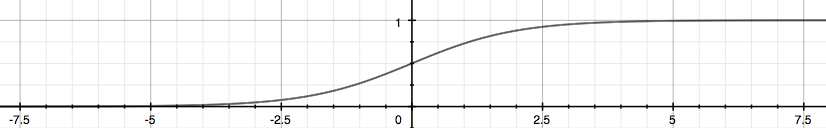The function g(z), shown here, maps any real number to the (0, 1) interval, making it useful for transforming an arbitrary-valued function into a function better suited for classification.

$h_θ(x)$ will give us the probability that our output is 1. For example, $h_θ(x)=0.7$ gives us a probability of 70% that our output is 1. Our probability that our prediction is 0 is just the complement of our probability that it is 1 (e.g. if probability that it is 1 is 70%, then the probability that it is 0 is 30%).

$h_\theta(x) = P(y=1 | x ; \theta) = 1 - P(y=0 | x ; \theta) \newline P(y = 0 | x;\theta) + P(y = 1 | x ; \theta) = 1$

$h_\theta (x) = g ( \theta^T x ) \newline \newline z = \theta^T x \newline g(z) = \dfrac{1}{1 + e^{-z}}$$h_θ(x)$ 将给我们输出为 1 的概率。例如，$h_θ(x) = 0.7$给我们将输出为 1 的概率为 70％。我们预测为 0 的概率就是它是 1 的概率的补码（例如，如果它是 1 的概率是 70％，那么它是 0 的概率是 30％）。

$h_\theta(x) = P(y=1 | x ; \theta) = 1 - P(y=0 | x ; \theta) \newline P(y = 0 | x;\theta) + P(y = 1 | x ; \theta) = 1$

## 三、决策边界¶

In order to get our discrete 0 or 1 classification, we can translate the output of the hypothesis function as follows:

$h_\theta(x) \geq 0.5 \rightarrow y = 1 \newline h_\theta(x) < 0.5 \rightarrow y = 0 \newline$

The way our logistic function g behaves is that when its input is greater than or equal to zero, its output is greater than or equal to 0.5:

$g(z) \geq 0.5 \newline when \; z \geq 0$

Remember.

$z=0, e^{0}=1 \Rightarrow g(z)=1/2\newline z \to \infty, e^{-\infty} \to 0 \Rightarrow g(z)=1 \newline z \to -\infty, e^{\infty}\to \infty \Rightarrow g(z)=0$

So if our input to g is $θ^TX$, then that means:

$h_\theta(x) = g(\theta^T x) \geq 0.5 \newline when \; \theta^T x \geq 0$

From these statements we can now say:

$\theta^T x \geq 0 \Rightarrow y = 1 \newline \theta^T x < 0 \Rightarrow y = 0 \newline$

The decision boundary is the line that separates the area where y = 0 and where y = 1. It is created by our hypothesis function.

Example:

$\theta = \begin{bmatrix}5 \newline -1 \newline 0\end{bmatrix} \newline y = 1 \; if \; 5 + (-1) x_1 + 0 x_2 \geq 0 \newline 5 - x_1 \geq 0 \newline - x_1 \geq -5 \newline x_1 \leq 5 \newline$

In this case, our decision boundary is a straight vertical line placed on the graph where $x1 = 5$, and everything to the left of that denotes $y = 1$, while everything to the right denotes $y = 0$.

Again, the input to the sigmoid function g(z) (e.g. $θ^TX$ doesn't need to be linear, and could be a function that describes a circle (e.g. $z = θ_0 + θ_1x_1^2 + θ_2x_2^2$) or any shape to fit our data.

$h_\theta(x) \geq 0.5 \rightarrow y = 1 \newline h_\theta(x) < 0.5 \rightarrow y = 0 \newline$

$g(z) \geq 0.5 \newline when \; z \geq 0$

$z=0, e^{0}=1 \Rightarrow g(z)=1/2\newline z \to \infty, e^{-\infty} \to 0 \Rightarrow g(z)=1 \newline z \to -\infty, e^{\infty}\to \infty \Rightarrow g(z)=0$

$h_\theta(x) = g(\theta^T x) \geq 0.5 \newline when \; \theta^T x \geq 0$

$\theta^T x \geq 0 \Rightarrow y = 1 \newline \theta^T x < 0 \Rightarrow y = 0 \newline$

Example:

$\theta = \begin{bmatrix}5 \newline -1 \newline 0\end{bmatrix} \newline y = 1 \; if \; 5 + (-1) x_1 + 0 x_2 \geq 0 \newline 5 - x_1 \geq 0 \newline - x_1 \geq -5 \newline x_1 \leq 5 \newline$

## 四、分类的代价函数¶

We cannot use the same cost function that we use for linear regression because the Logistic Function will cause the output to be wavy, causing many local optima. In other words, it will not be a convex function.

Instead, our cost function for logistic regression looks like:

$J(\theta) = \dfrac{1}{m} \sum_{i=1}^m \mathrm{Cost}(h_\theta(x^{(i)}),y^{(i)}) \newline \mathrm{Cost}(h_\theta(x),y) = -\log(h_\theta(x)) \; \text{if y = 1} \newline \mathrm{Cost}(h_\theta(x),y) = -\log(1-h_\theta(x)) \; \text{if y = 0}$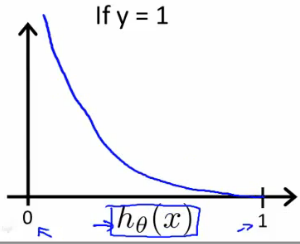Similarly, when $y = 0$, we get the following plot for $J(θ)$ vs $h_θ(x)$: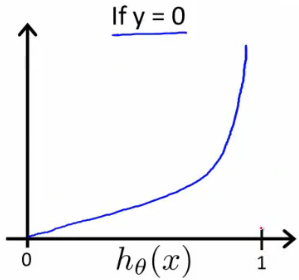$\mathrm{Cost}(h_\theta(x),y) = 0 \text{ if } h_\theta(x) = y \newline \mathrm{Cost}(h_\theta(x),y) \rightarrow \infty \text{ if } y = 0 \; \mathrm{and} \; h_\theta(x) \rightarrow 1 \newline \mathrm{Cost}(h_\theta(x),y) \rightarrow \infty \text{ if } y = 1 \; \mathrm{and} \; h_\theta(x) \rightarrow 0 \newline$

If our correct answer 'y' is 0, then the cost function will be 0 if our hypothesis function also outputs 0. If our hypothesis approaches 1, then the cost function will approach infinity.

If our correct answer 'y' is 1, then the cost function will be 0 if our hypothesis function outputs 1. If our hypothesis approaches 0, then the cost function will approach infinity.

Note that writing the cost function in this way guarantees that $J(θ)$ is convex for logistic regression.

$J(\theta) = \dfrac{1}{m} \sum_{i=1}^m \mathrm{Cost}(h_\theta(x^{(i)}),y^{(i)}) \newline \mathrm{Cost}(h_\theta(x),y) = -\log(h_\theta(x)) \; \text{if y = 1} \newline \mathrm{Cost}(h_\theta(x),y) = -\log(1-h_\theta(x)) \; \text{if y = 0}$$\mathrm{Cost}(h_\theta(x),y) = 0 \text{ if } h_\theta(x) = y \newline \mathrm{Cost}(h_\theta(x),y) \rightarrow \infty \text{ if } y = 0 \; \mathrm{and} \; h_\theta(x) \rightarrow 1 \newline \mathrm{Cost}(h_\theta(x),y) \rightarrow \infty \text{ if } y = 1 \; \mathrm{and} \; h_\theta(x) \rightarrow 0 \newline$

## 五、简化的成本函数和梯度下降¶

We can compress our cost function's two conditional cases into one case:

$\mathrm{Cost}(h_\theta(x),y) = - y \; \log(h_\theta(x)) - (1 - y) \log(1 - h_\theta(x))$

Notice that when y is equal to 1, then the second term $(1-y)\log(1-h_\theta(x))$ will be zero and will not affect the result. If y is equal to 0, then the first term $-y \log(h_\theta(x))$ will be zero and will not affect the result.

We can fully write out our entire cost function as follows:

$J(\theta) = - \frac{1}{m} \displaystyle \sum_{i=1}^m [y^{(i)}\log (h_\theta (x^{(i)})) + (1 - y^{(i)})\log (1 - h_\theta(x^{(i)}))]$

A vectorized implementation is:

$h = g(X\theta)\newline J(\theta) = \frac{1}{m} \cdot \left(-y^{T}\log(h)-(1-y)^{T}\log(1-h)\right)$

Remember that the general form of gradient descent is:

$Repeat \; \lbrace \newline \; \theta_j := \theta_j - \alpha \dfrac{\partial}{\partial \theta_j}J(\theta) \newline \rbrace$

We can work out the derivative part using calculus to get:

$Repeat \; \lbrace \newline \; \theta_j := \theta_j - \frac{\alpha}{m} \sum_{i=1}^m (h_\theta(x^{(i)}) - y^{(i)}) x_j^{(i)} \newline \rbrace$

Notice that this algorithm is identical to the one we used in linear regression. We still have to simultaneously update all values in $\theta$.

A vectorized implementation is:

$\theta := \theta - \frac{\alpha}{m} X^{T} (g(X \theta ) - \vec{y})$

$\mathrm{Cost}(h_\theta(x),y) = - y \; \log(h_\theta(x)) - (1 - y) \log(1 - h_\theta(x))$

$J(\theta) = - \frac{1}{m} \displaystyle \sum_{i=1}^m [y^{(i)}\log (h_\theta (x^{(i)})) + (1 - y^{(i)})\log (1 - h_\theta(x^{(i)}))]$

$h = g(X\theta)\newline J(\theta) = \frac{1}{m} \cdot \left(-y^{T}\log(h)-(1-y)^{T}\log(1-h)\right)$

$Repeat \; \lbrace \newline \; \theta_j := \theta_j - \alpha \dfrac{\partial}{\partial \theta_j}J(\theta) \newline \rbrace$

$Repeat \; \lbrace \newline \; \theta_j := \theta_j - \frac{\alpha}{m} \sum_{i=1}^m (h_\theta(x^{(i)}) - y^{(i)}) x_j^{(i)} \newline \rbrace$

$\theta := \theta - \frac{\alpha}{m} X^{T} (g(X \theta ) - \vec{y})$

## 六、高级优化¶

Conjugate gradient, BFGS, and L-BFGS are more sophisticated, faster ways to optimize θ that can be used instead of gradient descent. We suggest that you should not write these more sophisticated algorithms yourself (unless you are an expert in numerical computing) but use the libraries instead, as they're already tested and highly optimized. Octave provides them.

We first need to provide a function that evaluates the following two functions for a given input value $θ$:

$J(\theta) \newline \dfrac{\partial}{\partial \theta_j}J(\theta)$

We can write a single function that returns both of these:

1function [jVal, gradient] = costFunction(theta)2  jVal = [...code to compute J(theta)...];3  gradient = [...code to compute derivative of J(theta)...];4end

Then we can use octave's fminunc() optimization algorithm along with the optimset() function that creates an object containing the options we want to send to fminunc(). (Note: the value for MaxIter should be an integer, not a character string - errata in the video at 7:30)

1options = optimset('GradObj', 'on', 'MaxIter', 100);2initialTheta = zeros(2,1);3   [optTheta, functionVal, exitFlag] = fminunc(@costFunction, initialTheta, options);

We give to the function fminunc() our cost function, our initial vector of theta values, and the options object that we created beforehand.

共轭梯度(Conjugate gradient)BFGSL-BFGS 是优化 $θ$ 的更复杂，更快速的方法，可用于替代梯度下降。 我们建议您不要自己编写这些更复杂的算法（除非您是数值计算方面的专家），而应改用这些库，因为它们已经过测试和高度优化。 Octave 提供它们。

$J(\theta) \newline \dfrac{\partial}{\partial \theta_j}J(\theta)$

1function [jVal, gradient] = costFunction(theta)2  jVal = [...code to compute J(theta)...];3  gradient = [...code to compute derivative of J(theta)...];4end

1options = optimset('GradObj', 'on', 'MaxIter', 100);2initialTheta = zeros(2,1);3   [optTheta, functionVal, exitFlag] = fminunc(@costFunction, initialTheta, options);

## 七、多类别分类¶

Now we will approach the classification of data when we have more than two categories. Instead of y = {0,1} we will expand our definition so that y = {0,1...n}.

Since y = {0,1...n}, we divide our problem into n+1 (+1 because the index starts at 0) binary classification problems; in each one, we predict the probability that 'y' is a member of one of our classes.

$y \in \lbrace0, 1 ... n\rbrace \newline h_\theta^{(0)}(x) = P(y = 0 | x ; \theta) \newline h_\theta^{(1)}(x) = P(y = 1 | x ; \theta) \newline \cdots \newline h_\theta^{(n)}(x) = P(y = n | x ; \theta) \newline \mathrm{prediction} = \max_i( h_\theta ^{(i)}(x) )\newline$

We are basically choosing one class and then lumping all the others into a single second class. We do this repeatedly, applying binary logistic regression to each case, and then use the hypothesis that returned the highest value as our prediction.

The following image shows how one could classify 3 classes: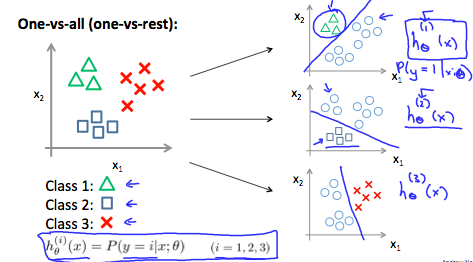To summarize:

Train a logistic regression classifier $h_θ(x)$ for each class to predict the probability that $y = i$.

To make a prediction on a new x, pick the class that maximizes $h_θ(x)$

$y \in \lbrace0, 1 ... n\rbrace \newline h_\theta^{(0)}(x) = P(y = 0 | x ; \theta) \newline h_\theta^{(1)}(x) = P(y = 1 | x ; \theta) \newline \cdots \newline h_\theta^{(n)}(x) = P(y = n | x ; \theta) \newline \mathrm{prediction} = \max_i( h_\theta ^{(i)}(x) )\newline$## 八、测验¶

1. Suppose that you have trained a logistic regression classifier, and it outputs on a new example $x$ a prediction $h_θ(x) = 0.2$. This means (check all that apply):
• a. Our estimate for $P(y=1|x;\theta)$ is 0.2.
• b. Our estimate for $P(y=0|x;\theta)$ is 0.2.
• a. Our estimate for $P(y=1|x;\theta)$ is 0.8.
• a. Our estimate for $P(y=0|x;\theta)$ is 0.8.

1. Suppose you have the following training set, and fit a logistic regression classifier $h_\theta(x) = g(\theta_0 + \theta_1x_1 + \theta_2 x_2)$.
$x1$ $x2$ $y$
1 0.5 0
1 1.5 0
2 1 1
3 1 0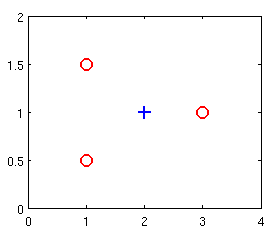Which of the following are true? Check all that apply.

• a. $J(\theta)$ will be a convex function, so gradient descent should converge to the global minimum.
• b. Adding polynomial features (e.g., instead using $h_\theta(x) = g(\theta_0 + \theta_1x_1 + \theta_2 x_2 + \theta_3 x_1^2 + \theta_4 x_1 x_2 + \theta_5 x_2^2)$ ) could increase how well we can fit the training data.
• c. The positive and negative examples cannot be separated using a straight line. So, gradient descent will fail to converge.
• d. Because the positive and negative examples cannot be separated using a straight line, linear regression will perform as well as logistic regression on this data.

1. For logistic regression, the gradient is given by $\frac{\partial}{\partial \theta_j} J(\theta) =\frac{1}{m}\sum_{i=1}^m{ (h_\theta(x^{(i)}) - y^{(i)}) x_j^{(i)}}$. Which of these is a correct gradient descent update for logistic regression with a learning rate of $α$? Check all that apply.
• a. $\theta := \theta - \alpha \frac{1}{m} \sum_{i=1}^m{ \left(\theta^T x - y^{(i)}\right) x^{(i)}}$.
• b. $\theta_j := \theta_j - \alpha \frac{1}{m} \sum_{i=1}^m{ (h_\theta(x^{(i)}) - y^{(i)}) x_j^{(i)}}$ (simultaneously update for all $j$).
• c. $\theta_j := \theta_j - \alpha \frac{1}{m} \sum_{i=1}^m{ (h_\theta(x^{(i)}) - y^{(i)}) x^{(i)}}$ (simultaneously update for all $j$).
• d. $\theta_j := \theta_j - \alpha \frac{1}{m} \sum_{i=1}^m{ \left(\frac{1}{1 + e^{-\theta^T x^{(i)}}} - y^{(i)}\right) x_j^{(i)}}$ (simultaneously update for all $j$).

1. Which of the following statements are true? Check all that apply.
• a. Since we train one classifier when there are two classes, we train two classifiers when there are three classes (and we do one-vs-all classification).
• b. The one-vs-all technique allows you to use logistic regression for problems in which each $y^{(i)}$ comes from a fixed, discrete set of values.
• c. The cost function $J(\theta)$ for logistic regression trained with $m \geq 1$ examples is always greater than or equal to zero.
• d. For logistic regression, sometimes gradient descent will converge to a local minimum (and fail to find the global minimum). This is the reason we prefer more advanced optimization algorithms such as fminunc (conjugate gradient/BFGS/L-BFGS/etc).

1. Suppose you train a logistic classifier $h_\theta(x) = g(\theta_0 + \theta_1x_1 + \theta_2 x_2)$. Suppose $\theta_0 = - 6, \theta_1 = 1, \theta_2 = 0$. Which of the following figures represents the decision boundary found by your classifier?
• a. Figure: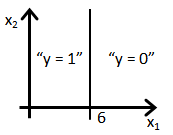• b. Figure: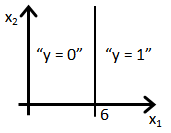• c. Figure: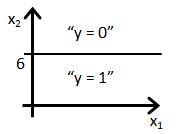• d. Figure: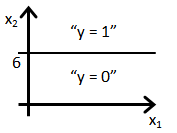1. 假设您已经训练了逻辑回归分类器，并且在新示例 $x$ 上输出了预测 $h_θ(x) = 0.2$。 这意味着（多选）
• a. 我们对 $P(y=1|x;\theta)$ 的估计为 0.2。
• b. 我们对 $P(y=0|x;\theta)$ 的估计为 0.2。
• c. 我们对 $P(y=1|x;\theta)$ 的估计为 0.8。
• d. 我们对 $P(y=0|x;\theta)$ 的估计为 0.8。

1. 假设您具有以下训练集，并拟合逻辑回归分类器 $h_\theta(x) = g(\theta_0 + \theta_1x_1 + \theta_2 x_2)$
$x1$ $x2$ $y$
1 0.5 0
1 1.5 0
2 1 1
3 1 0• a. $J(\theta)$ 将是一个凸函数，因此梯度下降应收敛到全局最小值。
• b. 添加多项式特征 (例如，改为使用 $h_\theta(x) = g(\theta_0 + \theta_1x_1 + \theta_2 x_2 + \theta_3 x_1^2 + \theta_4 x_1 x_2 + \theta_5 x_2^2)$) 可能会增加我们拟合训练数据的能力。
• c. 正例和负例不能使用直线分开。因此，梯度下降将无法收敛。
• d. 因为正和负的例子不能用直线分开，线性回归将执行以及该数据逻辑回归。

1. 对于逻辑回归，梯度为 $\frac{\partial}{\partial \theta_j} J(\theta) =\frac{1}{m}\sum_{i=1}^m{ (h_\theta(x^{(i)}) - y^{(i)}) x_j^{(i)}}$。 以下哪项是学习速率为 $α$ 的逻辑回归的正确梯度下降更新？（多选）
• a. $\theta := \theta - \alpha \frac{1}{m} \sum_{i=1}^m{ \left(\theta^T x - y^{(i)}\right) x^{(i)}}$.
• b. $\theta_j := \theta_j - \alpha \frac{1}{m} \sum_{i=1}^m{ (h_\theta(x^{(i)}) - y^{(i)}) x_j^{(i)}}$